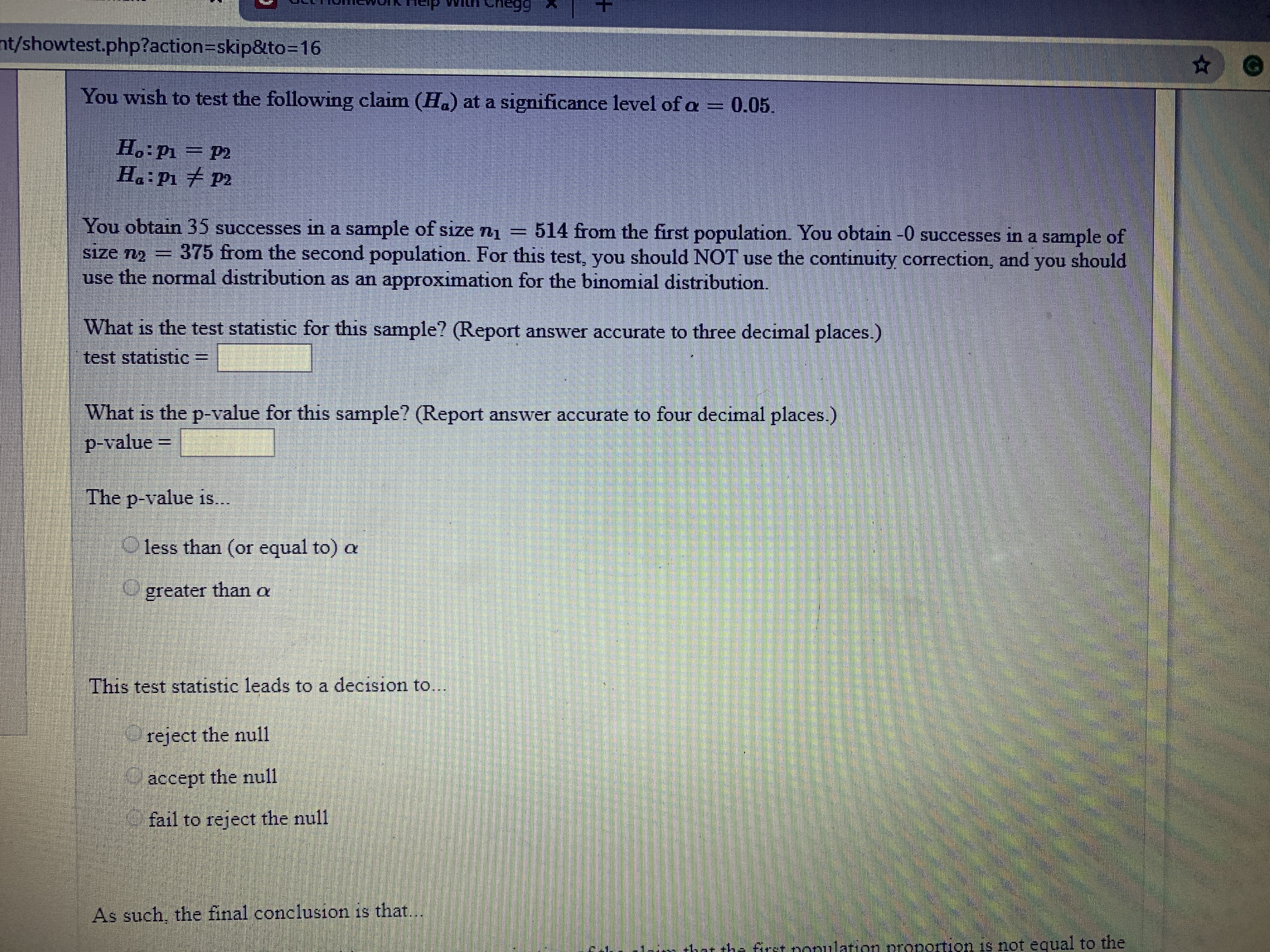# itn eneee nt/showtest.php?action=skip&to-16 You wish to test the following claim (Ha) at a significance level of a 0.05. H.:Pi P2 H: Pi P2 You obtain 35 successes in a sample of size n1 514 from the first population. You obtain -0 successes in a sample of 375 from the second population. For this test, you should NOT use the continuity correction, and you should S1ze 12 use the normal distribution as an approximation for the binomial distribution. What is the test statistic for this sample? (Report answer accurate to three decimal places.) test statistic What is the p-value for this sample? (Report answer accurate to four decimal places.) P-value The p-value is.. 1ess than (or equal to) a greater than a This test statistic leads to a decision to.. reject the null accept the null fail to reject the null As such, the final conclusion is that. hot the first nonulation proportion 1s not equal to the

Questionhelp_outlineImage Transcriptioncloseitn eneee nt/showtest.php?action=skip&to-16 You wish to test the following claim (Ha) at a significance level of a 0.05. H.:Pi P2 H: Pi P2 You obtain 35 successes in a sample of size n1 514 from the first population. You obtain -0 successes in a sample of 375 from the second population. For this test, you should NOT use the continuity correction, and you should S1ze 12 use the normal distribution as an approximation for the binomial distribution. What is the test statistic for this sample? (Report answer accurate to three decimal places.) test statistic What is the p-value for this sample? (Report answer accurate to four decimal places.) P-value The p-value is.. 1ess than (or equal to) a greater than a This test statistic leads to a decision to.. reject the null accept the null fail to reject the null As such, the final conclusion is that. hot the first nonulation proportion 1s not equal to the fullscreen

### Want to see this answer and more?

Experts are waiting 24/7 to provide step-by-step solutions in as fast as 30 minutes!*

*Response times may vary by subject and question complexity. Median response time is 34 minutes for paid subscribers and may be longer for promotional offers.
Tagged in
Math
Statistics

### Hypothesis Testing